How Cheenta works to ensure student success?
Explore the Back-Story

# Relation Mapping (IIT JAM 2014)## Question 33 - Relation-Mapping (IIT JAM 2014)

A beautiful problem involving the concept of relation-mapping from IIT JAM 2014.

Let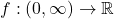be a differentiable function such that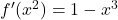for all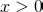and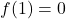. Then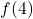equals

••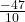•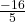•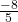### Key Concepts

Relation/Mapping

Differentiation

Integration

Answer: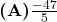.

Question 33 (IIT JAM 2014)

Real Analysis (Willy)

## Try with Hints

The above problem can be done in many ways we will try to solve this by the simplest method.

Now, as the function is given asSo first try to change this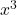into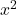. Try this. It's very easy !!!

To changeintowe can easily do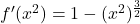Now we have to find the value ofso we have to change the second degree term, i.e.,into some linear form. Can you cook this up ???

Let us assume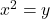i.e.,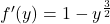Now you know from previous knowledge that integration is also known as anti-derivative. Socan be changed into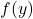by integrating it with respect to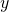. Try to do this integration and we are half way done !!!

On integrating both side w.r.twe get :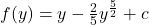, (where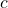is a integrating constant.)

Now we find the value toWe know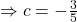i.e.,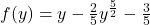Can you find the answer now ?

Now simply, puttingwe get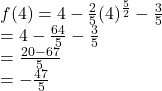## Question 33 - Relation-Mapping (IIT JAM 2014)

A beautiful problem involving the concept of relation-mapping from IIT JAM 2014.

Letbe a differentiable function such thatfor alland. Thenequals

••••### Key Concepts

Relation/Mapping

Differentiation

Integration

Answer:.

Question 33 (IIT JAM 2014)

Real Analysis (Willy)

## Try with Hints

The above problem can be done in many ways we will try to solve this by the simplest method.

Now, as the function is given asSo first try to change thisinto. Try this. It's very easy !!!

To changeintowe can easily doNow we have to find the value ofso we have to change the second degree term, i.e.,into some linear form. Can you cook this up ???

Let us assumei.e.,Now you know from previous knowledge that integration is also known as anti-derivative. Socan be changed intoby integrating it with respect to. Try to do this integration and we are half way done !!!

On integrating both side w.r.twe get :, (whereis a integrating constant.)

Now we find the value toWe knowi.e.,Can you find the answer now ?

Now simply, puttingwe get## Subscribe to Cheenta at Youtube

This site uses Akismet to reduce spam. Learn how your comment data is processed.

### Knowledge Partner